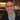# Format a US SSN input while typing with Javascript.

January 10, 2022.Written by Thomas Duffy

## Intro

We’ll create an input field that formats a US social security number (SSN) with Javascript from scratch in this post. This post will not be library-specific, so; I will write the examples in vanilla JS and HTML.

## Example of what we’ll buildWe’re going to start by creating the HTML structure and input element for the SSN. We will also add an id attribute of “ssn” to hook up our formatting function later.

``````<!DOCTYPE html>
<html>
<body>
<input id="ssn" />
</body>
</html>``````

##Add the handler and script tag for some JS

Next, we will add the onkeydown event handler on the input element to call the function for formatting the SSN while the user is typing. We’ll also add an empty script tag to add our formatting function.

``````<!DOCTYPE html>
<html>
<body>
<input onkeydown="ssnFormatter()" id="ssn" />
</body>
<script></script>
</html>``````

##Create onkeydown handler to hook up format function

Now that we have our script tag and input elements, we can start by writing the `ssnFormater` function. We will use this function to hook onto the input element, format the value, and reset the input value to the formatted version. This function should be inside the `script` tag we added in the step above.

``````function ssnFormatter() {
// grab the value of what the user is typing into the input
const inputField = document.getElementById('ssn');
// next, we're going to format this input with the `formatSSN` function, which we'll write next.
const formatedValue = formatSSN(inputField.value);
// Then we'll set the value of the inputField to the formattedValue we generated with the formatSSN function
inputField.value = formattedInputValue;
}``````

##Write custom format function to validate and format social security number (SSN) input

So the `ssnFormater` function hooks onto the input element, passes the value into our future custom format function, and then sets the input elements value to the new formatted value. Now, let’s write our custom `formatSSN` function.

``````function formatSSN(value) {
// if input value is falsy eg if the user deletes the input, then just return
if (!value) return value;

// clean the input for any non-digit values.
const ssn = value.replace(/[^\d]/g, '');

// ssnLength is used to know when to apply our formatting for the ssn
const ssnLength = ssn.length;

// we need to return the value with no formatting if its less than four digits
if (ssnLength < 4) return ssn;

// if ssnLength is greater than 4 and less the 6 we start to return
// the formatted number
if (ssnLength < 6) {
return `\${ssn.slice(0, 3)}-\${ssn.slice(3)}`;
}

// finally, if the ssnLength is greater then 6, we add the last
// bit of formatting and return it.
return `\${ssn.slice(0, 3)}-\${ssn.slice(3, 5)}-\${ssn.slice(5, 8)}`;
}``````

##Final code for phone number formatting input

Ok, now our final markup should look like something below.

``````<!DOCTYPE html>
<html>
<body>
<input onkeydown="ssnFormatter()" id="ssn" />

<script>
function formatSSN(value) {
if (!value) return value;
const ssn = value.replace(/[^\d]/g, '');
const ssnLength = ssn.length;

if (ssnLength < 4) return ssn;

if (ssnLength < 6) {
return `\${ssn.slice(0, 3)}-\${ssn.slice(3)}`;
}

return `\${ssn.slice(0, 3)}-\${ssn.slice(3, 5)}-\${ssn.slice(5, 8)}`;
}

function ssnFormatter() {
const inputField = document.getElementById('ssn');
const formattedInputValue = formatSSN(inputField.value);
inputField.value = formattedInputValue;
}
</script>
</body>
</html>``````

##Conclusion on formatting and validating a social security number (SSN) with Javascript.

I hope you enjoyed this post on formatting and validating a social security number (SSN) using vanilla Javascript. If you like this post, please sign up for my newsletter, where you can be notified when I drop new posts related to frontend web development.Written by Thomas Duffy

### Popular Posts9 ways you can be a better programmer